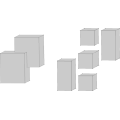CGAL 4.8.2 - Convex Decomposition of Polyhedra
Convex Decomposition of Polyhedra ReferencePeter Hachenberger
This packages provides a function for decomposing a bounded polyhedron into convex sub-polyhedra. The decomposition yields $$O(r^2)$$ convex pieces, where $$r$$ is the number of edges, whose adjacent facets form an angle of more than 180 degrees with respect to the polyhedron's interior. This bound is worst-case optimal.

Introduced in: CGAL 3.5
BibTeX: cgal:h-emspe-16b
Windows Demo: Operations on Polyhedra
Common Demo Dlls: dlls

## Functions

• CGAL::convex_decomposition_3(Nef_polyhedron_3& N)

## Functions

template<typename Nef_polyhedron >
void CGAL::convex_decomposition_3 (Nef_polyhedron &N)
The function convex_decomposition_3() inserts additional facets into the given Nef_polyhedron_3 N, such that each bounded marked volume (the outer volume is unbounded) is subdivided into convex pieces. More...

## Function Documentation

template<typename Nef_polyhedron >
 void CGAL::convex_decomposition_3 ( Nef_polyhedron & N)

The function convex_decomposition_3() inserts additional facets into the given Nef_polyhedron_3 N, such that each bounded marked volume (the outer volume is unbounded) is subdivided into convex pieces.

The modified polyhedron represents a decomposition into $$O(r^2)$$ convex pieces, where $$r$$ is the number of edges that have two adjacent facets that span an angle of more than 180 degrees with respect to the interior of the polyhedron.

The worst-case running time of our implementation is $$O(n^2r^4\sqrt{nr^2}\log{(nr)})$$, where $$n$$ is the complexity of the polyhedron (the complexity of a Nef_polyhedron_3 is the sum of its Vertices, Halfedges and SHalfedges) and $$r$$ is the number of reflex edges.

Precondition
The polyhedron N is bounded. Otherwise, the outer volume is ignored.
Postcondition
If the polyhedron N is non-convex, it is modified to represent the convex decomposition. If N is convex, it is not modified.
CGAL::Nef_polyhedron_3<Traits>
#include <CGAL/convex_decomposition_3.h>